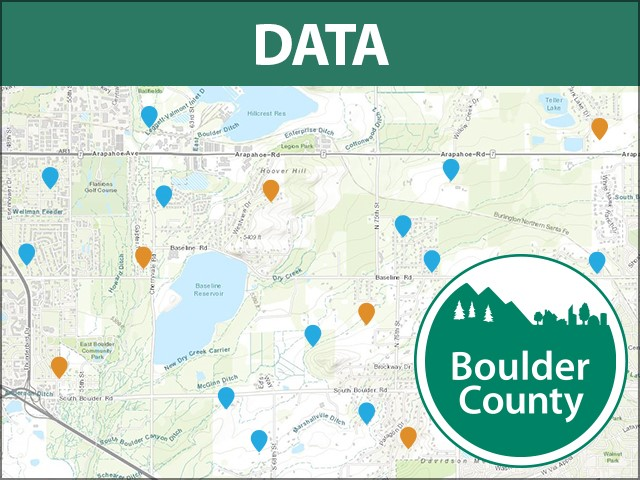### Description

more
FEMA DFIRM (Digital Flood Insurance Rate Map) floodplain effective December 18, 2012, created for Boulder County and all municipalities within under the direction of the FEMA Map Modernization project. This data supercedes all other flood data.Per FEMA: Data included on this CD-ROM represents Final Flood Insurance Rate Map (FIRM) data that has been published as effective FIRM or DFIRM information. The data are the official and legal representation of the effective flood zones.Further edits include two LOMRs dated after 12/18/12.Boulder County added a field, "Regulating Entity" to distinguish this from Boulder County regulatory floodplain.

### Dataset Attributes

• OBJECTID
Number
• DFIRM_ID
Text
{"value"=>"08013C", "count"=>1422} ()
• VERSION_ID
Text
{"value"=>"2.3.3.2", "count"=>895} (), {"value"=>"1.1.1.0", "count"=>527} ()
• FLD_AR_ID
Text
{"value"=>" ", "count"=>1955} (), {"value"=>"10525", "count"=>489} (), {"value"=>"8504", "count"=>97} (), {"value"=>"8816", "count"=>84} (), {"value"=>"9856", "count"=>64} (), {"value"=>"10148", "count"=>42} (), {"value"=>"9642", "count"=>33} (), {"value"=>"8889", "count"=>27} (), {"value"=>"10088", "count"=>25} (), {"value"=>"8422", "count"=>25} ()
• STUDY_TYP
Text
{"value"=>"NP", "count"=>527, "code"=>"NP"} (), {"value"=>"SFHAs WITH LOW FLOOD RISK", "count"=>521, "code"=>"SFHAs WITH LOW FLOOD RISK"} (), {"value"=>"SFHAs WITH HIGH FLOOD RISK", "count"=>366, "code"=>"SFHAs WITH HIGH FLOOD RISK"} (), {"value"=>"SFHAs WITH MEDIUM FLOOD RISK", "count"=>8, "code"=>"SFHAs WITH MEDIUM FLOOD RISK"} ()
• FLD_ZONE
Text
{"value"=>"X", "count"=>4672, "code"=>"X"} (), {"value"=>"AE", "count"=>1636, "code"=>"AE"} (), {"value"=>"A", "count"=>85, "code"=>"A"} (), {"value"=>"AO", "count"=>40, "code"=>"AO"} (), {"value"=>"AH", "count"=>15, "code"=>"AH"} ()
• ZONE_SUBTY
Text
{"value"=>"AREA OF MINIMAL FLOOD HAZARD", "count"=>2640, "code"=>"2000"} (), {"value"=>"0.2 PCT ANNUAL CHANCE FLOOD HAZARD", "count"=>2010, "code"=>"0500"} (), {"value"=>"FLOODWAY", "count"=>174, "code"=>"1100"} (), {"value"=>"AREA WITH REDUCED FLOOD RISK DUE TO LEVEE", "count"=>20, "code"=>"1000"} (), {"value"=>"1 PCT DEPTH LESS THAN 1 FOOT", "count"=>2, "code"=>"0400"} ()
• SFHA_TF
Text
{"value"=>"F", "count"=>2729, "code"=>"F"} (), {"value"=>"T", "count"=>1774, "code"=>"T"} ()
• STATIC_BFE
Number
-9999 to 5529
• V_DATUM
Text
{"value"=>"NP", "count"=>18, "code"=>"NP"} (), {"value"=>"NAVD88", "count"=>9, "code"=>"NAVD88"} ()
• DEPTH
Number
-9999 to 3
• LEN_UNIT
Text
{"value"=>"FEET", "count"=>49, "code"=>"FEET"} ()
• VELOCITY
Number
-9999 to 0
• VEL_UNIT
Text
• AR_REVERT
Text
• AR_SUBTRV
Text
{"value"=>" ", "count"=>1422, "code"=>" "} ()
• BFE_REVERT
Number
-9999 to 0
• DEP_REVERT
Number
-9999 to 0
• DUAL_ZONE
Text
{"value"=>"F", "count"=>954, "code"=>"F"} (), {"value"=>" ", "count"=>451, "code"=>" "} (), {"value"=>"U", "count"=>17, "code"=>"U"} ()
• SOURCE_CIT
Text
{"value"=>"STUDY2", "count"=>2245} (), {"value"=>"STUDY1", "count"=>1662} (), {"value"=>"STUDY8", "count"=>895} (), {"value"=>"STUDY6", "count"=>282} (), {"value"=>"LOMC58", "count"=>173} (), {"value"=>"LOMC30", "count"=>171} (), {"value"=>"LOMC57", "count"=>128} (), {"value"=>"LOMC33", "count"=>99} (), {"value"=>"STUDY4", "count"=>60} (), {"value"=>"LOMC40", "count"=>50} ()
• REGULATING_ENTITY
Text
{"value"=>"FEMA", "count"=>6448} ()
• SHAPE
Number
• SHAPE.STArea()
Number
• SHAPE.STLength()
Number

• By on June 1, 2017
• Updated 3 months ago

### Related Datasets

• Floodplain - BC RegulatedFloodplain - BC Regulated
• Mobile HomesMobile Homes
• Road Closures TEST• Fourmile Fire Perimeter - September 13th 2010